## Binary

The Base 2 method of counting in which only the digits 0 and 1 are used. In this Base, the number 1011 equals. This Base is used in computers, since all numbers can be simply represented as a string of electrically pulsed ons and offs. A Negative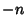is most commonly represented as the complement of the Positive number, so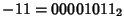would be written as the complement of, or 11110101. This allows addition to be carried out with the usual carrying and the left-most digit discarded, so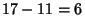gives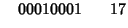The number of timesa given binary numberis divisible by 2 is given by the position of the firstcounting from the right. For example,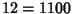is divisible by 2 twice, and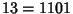is divisible by 2 0 times.

Unfortunately, the storage of binary numbers in computers is not entirely standardized. Because computers store information in 8-bit bytes (where a bit is a single binary digit), depending on the word size'' of the machine, numbers requiring more than 8 bits must be stored in multiple bytes. The usual FORTRAN77 integer size is 4 bytes long. However, a number represented as (byte1 byte2 byte3 byte4) in a VAX would be read and interpreted as (byte4 byte3 byte2 byte1) on a Sun. The situation is even worse for floating point (real) numbers, which are represented in binary as a Mantissa and Characteristic, and worse still for long (8-byte) reals!

Binary multiplication of single bit numbers (0 or 1) is equivalent to the And operation, as can be seen in the following Multiplication Table.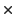0 1 0 0 0 1 0 1

References

Lauwerier, H. Fractals: Endlessly Repeated Geometric Figures. Princeton, NJ: Princeton University Press, pp. 6-9, 1991.

Pappas, T. Computers, Counting, & Electricity.'' The Joy of Mathematics. San Carlos, CA: Wide World Publ./Tetra, pp. 24-25, 1989.

Press, W. H.; Flannery, B. P.; Teukolsky, S. A.; and Vetterling, W. T. Error, Accuracy, and Stability'' and Diagnosing Machine Parameters.'' §1.2 and §20.1 in Numerical Recipes in FORTRAN: The Art of Scientific Computing, 2nd ed. Cambridge, England: Cambridge University Press, pp. 18-21, 276, and 881-886, 1992.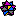Weisstein, E. W. Bases.'' Mathematica notebook Bases.m.